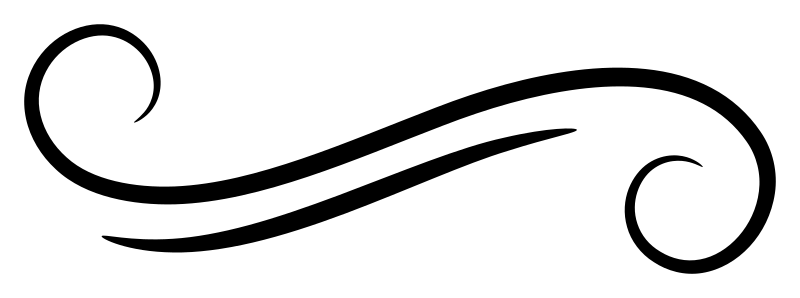# Derivable type classes

September 2000Derivable type classesDerivable type classes

This paper presents an approach for introducing generic programming to the Haskell language. Since Haskell’s first specification, it has always been possible to let the compiler automatically generate “obvious” instances — however, this feature known as stock deriving supported only a handful of pre-defined classes. This new idea would allow users who write their own typeclasses to also specify implicit implementations that work for any datatype.

The main contribution generic functions, a new sort of default class method that can describe very generally how to handle sum and product datatypes. The paper’s first example shows how `Eq` deriving could be defined by writing `(==)` as a generic function:

``````class Eq t where
(==), (/=) :: t -> t -> Bool

(==) { 1 } Unit Unit = True
(==) { a + b } (Inl x1) (Inl x2) = True
(==) { a + b } (Inr y1) (Inr y2) = True
(==) { a + b } _ _ = False
(==) { a * b } (x1 :*: y1) (x2 :*: y2) =
(x1 == x2) && (y1 == y2)

(/=) x1 x2 = not (x1 == x2)``````

This feature first appears in GHC 5.00.

Authors: Ralf Hinze and Simon Peyton JonesJoin Type Classes for courses and projects to get you started and make you an expert in FP with Haskell.